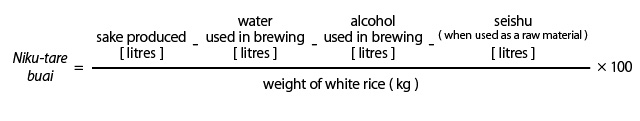# Shuka-ritsu

【English】
Rate of sake yield

This is the ratio of sake produced to the weight of white rice used. It is necessary to establish this value for various reasons related to the production process, and this is done by various methods. It can be expressed in terms of: 1) niku-tare buai; 2) alcohol yield per 1000 kg white rice; 3) gen ekisu (gen-extract) yield rate.

The first is the most widely used, and represents the amount of sake produced in relation to the quantity of actual white rice used. This value is calculated by the following formula, subtracting all other raw materials used except white rice from the volume of sake produced.The starch in white rice is converted to alcohol after being broken down into saccharides. The yield rate as calculated with sugars in addition to the quantity of alcohol produced is known as gen ekisu and is calculated as follows.

Gen ekisu = [ (S – A) × 260 + 0.21 ] + ( alcohol content ) × 0.7947 × 2

Note: S represents specific gravity, and the value A is alcohol calculated as specific gravity.